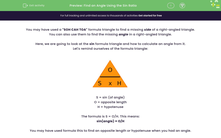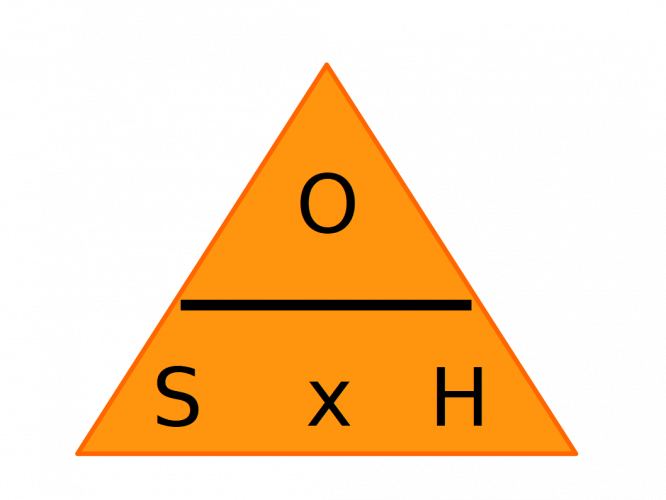# Find an Angle Using the Sin Ratio

In this worksheet, students will use the Sin ratio to find the missing angle of a triangle.Key stage:  KS 4

Year:  GCSE

GCSE Subjects:   Maths

GCSE Boards:   AQA, Eduqas, Pearson Edexcel, OCR,

Curriculum topic:   Geometry and Measures, Mensuration

Curriculum subtopic:   Mensuration and Calculation Triangle Mensuration

Popular topics:   Angles worksheets, Geometry worksheets

Difficulty level:#### Worksheet Overview

You may have used a "SOH CAH TOA" formula triangle to find a missing side of a right-angled triangle.

You can also use them to find the missing angle in a right-angled triangle.

Here, we are going to look at the sin formula triangle and how to calculate an angle from it.

Let's remind ourselves of the formula triangle:S = sin (of angle)
O = opposite length
H = hypotenuse

The formula is S = O/H. This means:

sin(angle) = O/H

You may have used formula this to find an opposite length or hypotenuse when you had an angle.

If you are finding an angle, you substitute the lengths into the same formula - you just need to then use a function known as inverse sin to calculate it (this is written as sin-1). On most calculators, this is accessed by pressing SHIFT and then sin.

So, the formula for finding an angle with an opposite and hypotenuse is:

angle = sin-1(O/H)

Let's give it a go...

IMPORTANT NOTE: Make sure your calculator is set to degrees. You can tell as a "D" appears at the top of the calculator screen.

Example

Find angle x in this triangle.1. Label the triangle.

2. Identify the two sides you want to use. If you want to find the angle using sin, you will need the hypotenuse H, and the length of the opposite side, O.

3. Substitute the lengths into the formula S = O ÷ H to calculate sin x.

9 ÷ 12 = 0.75

sin x = 0.75

4. Finally, use the inverse sin button (sin -1) on your calculator on this value.

x = sin-1(0.75) = 48.6° (to 1 decimal place)

If you follow these steps, you should be able to find the angle in a right angled triangle when you have O and H.

Have a go at this activity and practise this skill!

### What is EdPlace?

We're your National Curriculum aligned online education content provider helping each child succeed in English, maths and science from year 1 to GCSE. With an EdPlace account you’ll be able to track and measure progress, helping each child achieve their best. We build confidence and attainment by personalising each child’s learning at a level that suits them.

Get started